Bybit Learn
Intermediate
Jun 22, 2021

# How to Use Fibonacci Retracement Tools to Trade Bitcoin

Fibonacci retracement levels are a popular tool used by traders to identify turning points in cryptocurrency prices. Without this tool, traders might enter into Bitcoin trades without confidence, which can lead to unnecessary losses.

While the Fibonacci retracement tool is commonly used in the traditional stock or forex market, you’ll be surprised to know that it works wonderfully for cryptocurrency markets too.

In this guide, we’ll decipher the technicalities of this tool. In the end, you’ll learn how you can use it to find critical levels on a trading chart.

## What Are Fibonacci Retracement Levels?

Fibonacci retracements are hidden levels of the horizontal lines of support and resistance where Bitcoin prices may potentially reverse. The main levels are 23.6%, 38.2%, 61.8% and 78.6%.

The Fibonacci retracement is derived from Fibonacci sequence, which occurs in nature and mathematics, introduced by an Italian mathematician. They are used in finance by traders when analyzing a price chart to determine potential turning points.

## What Does the Ratio Represent?

The Fibonacci retracement is expressed as a percentage and is applied to a previous trend. The retracement indicates how much of the previous trend is likely to be corrected before a resumption of the older trend. The Fibonacci retracement tool converts the percentage into Bitcoin’s price, identifying where the market may pivot and change trends.

There are four main retracement levels where a reversal may begin.

• 23.6%
• 38.2%
• 61.8%
• 78.6%

These key Fibonacci ratios seem like random figures, but they are not. They are deeply rooted in an organic number sequence discovered by humans over 700 years ago.

## Who Created Fibonacci Retracement?

Fibonacci retracement percentages were created from the Fibonacci number sequence. Leonardo de Pisa discovered and shared this number sequence in his book, Liber Abaci (The Book of Numbers), published in 1202.

The Fibonacci number sequence looks like this:

1, 1, 2, 3, 5, 8, 13, 21, 34, 55, 89, 144, 233, 377, 610 …

The next number in the sequence is always the sum of the previous two numbers. The Fibonacci number sequence comes alive when you look at the ratio between adjacent numbers in the sequence.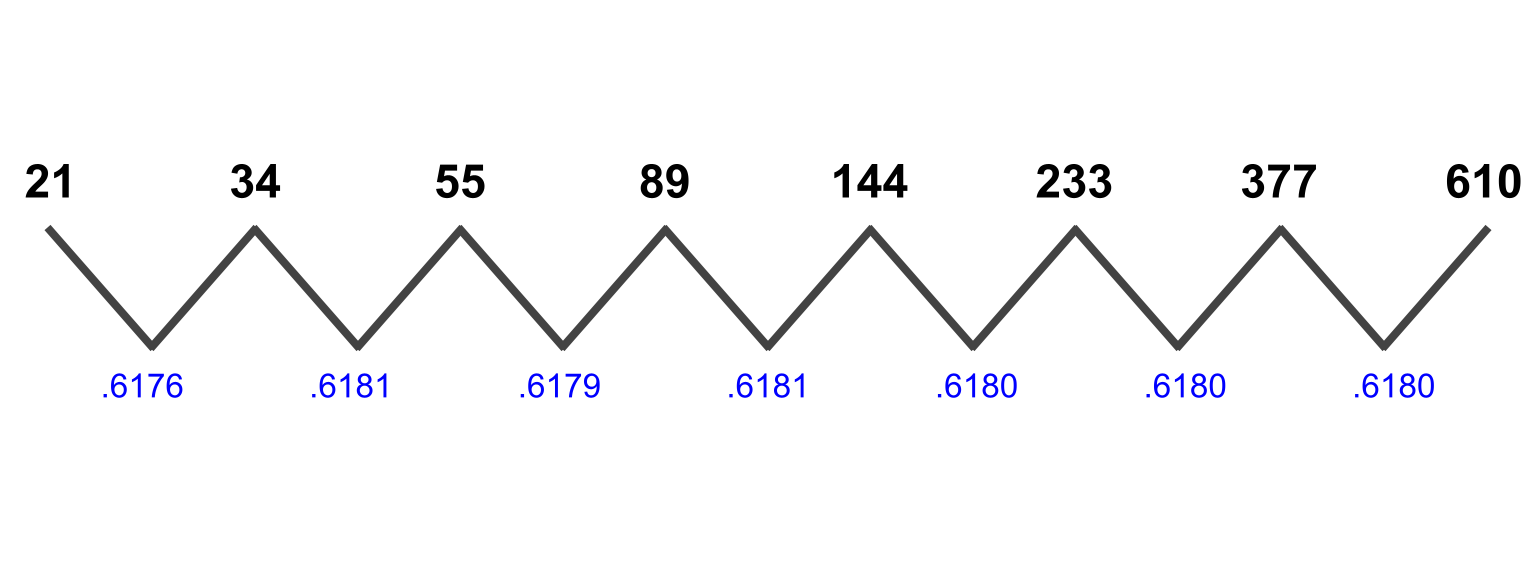The Fibonacci number sequence.

For example, 21 divided by 34 equals .6176. Notice how the ratio of two adjacent numbers moves closer to .618 as the number sequence extends to the right. The .618 ratio translates to a percentage of 61.8%, which is one of the key levels.

We can apply this methodology again, dividing by every other number in the sequence.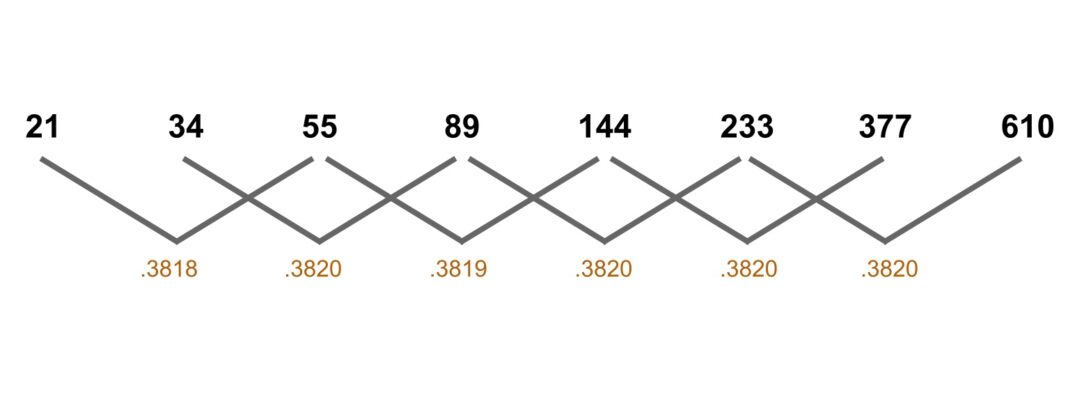The second Fibonacci retracement number.

In the second example, 21 divided by 55 equals .3818. A ratio of every two numbers in the sequence drives toward .382, or 38.2%, the second key level.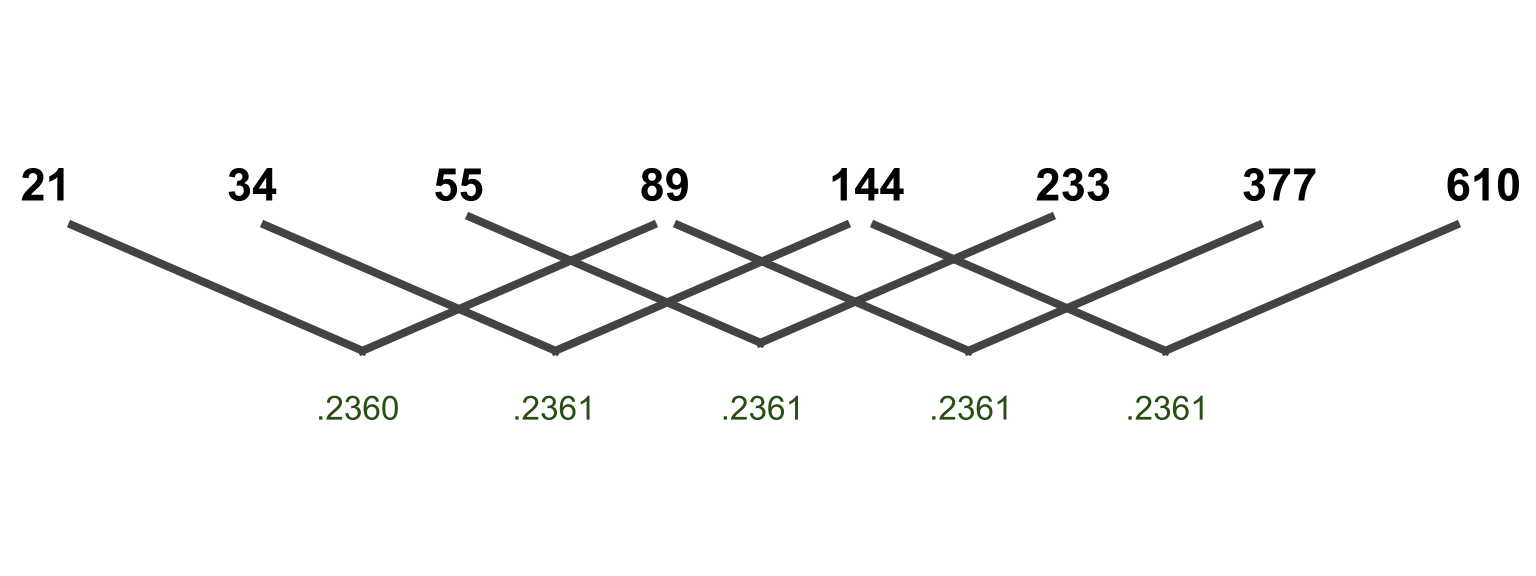The third Fibonacci retracement number.

If we apply this methodology a third time, dividing by every third number in the sequence, we find 21 divided by 89 equals .2360. As this number sequence moves to the right, the ratio moves toward .236, or 23.6%. This is the third Fibonacci retracement number.

The fourth and final level is 78.6%, or .786 — which is the square root of .618, our first key level.

## Fibonacci Retracement Ratio Calculation

To apply the tool correctly, we first need a completed trend. The Fibonacci retracement tool will measure the length of the trend, then divide out the four key levels.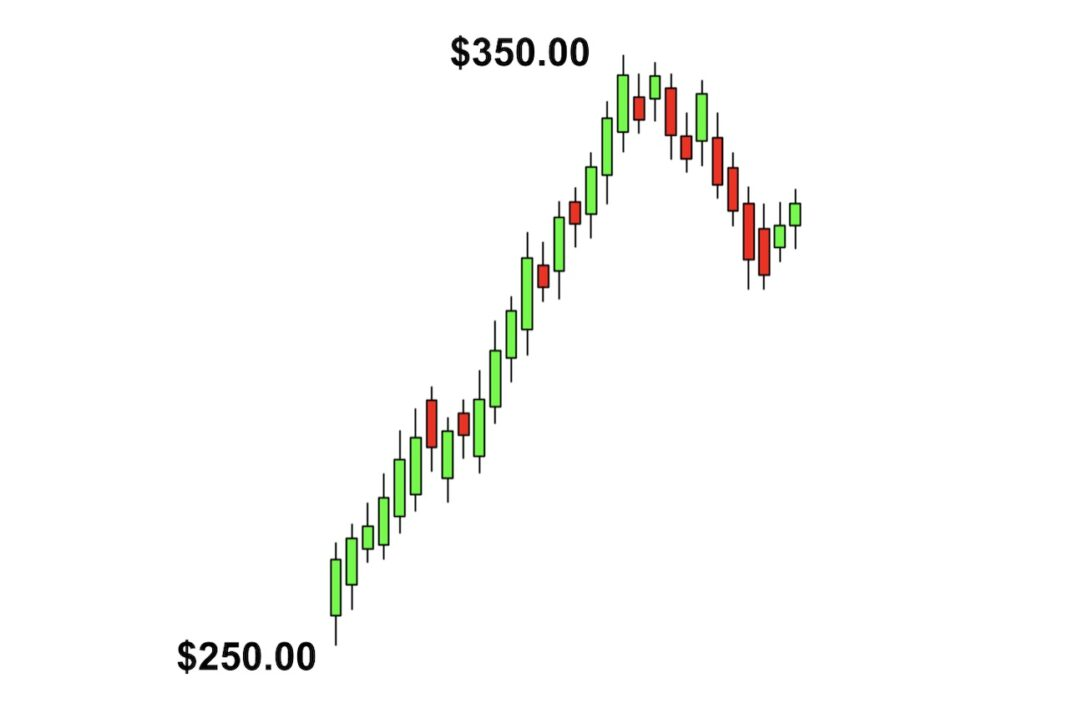In the example above, let’s assume the uptrend started at \$250 and topped out at \$350, for a gain of \$100.

The four ratios of 23.6%, 38.2%, 61.8%, and 78.6% are applied to the size of the trend, which is \$100.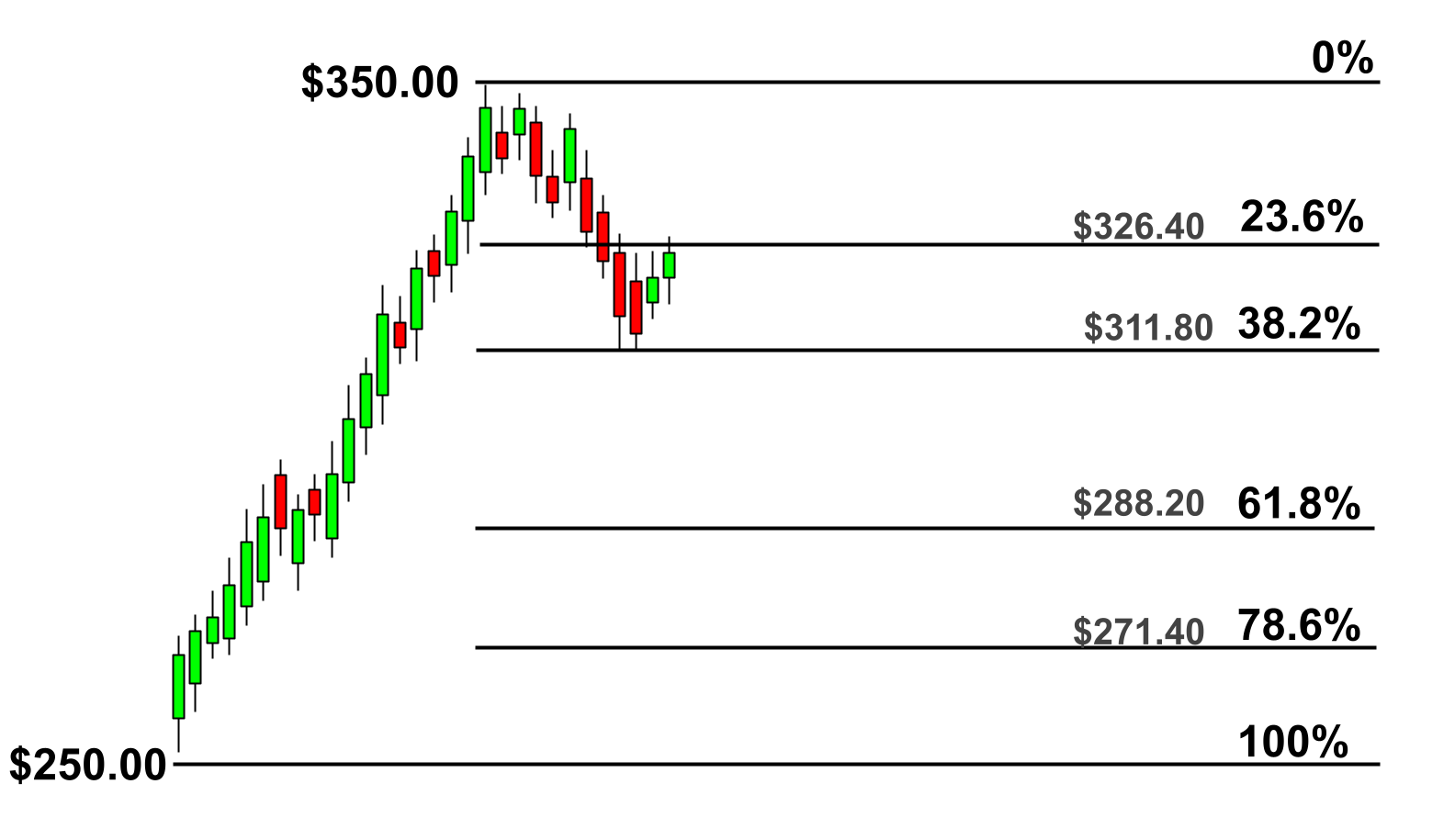This first retracement level at 23.6% will correct \$23.60 from the price high (\$100 uptrend x 23.6% = \$23.60). \$350 minus \$23.60 = \$326.40.

The second retracement level at 38.2% will correct \$38.20 from the \$350 high (\$100 uptrend x 38.2% = \$38.20).

The calculations are similar for the third and fourth levels, using 61.8% and 78.6% as the multipliers.

After these calculations have been made, we have four price zones where a correction of the downtrend may stop and reverse back into an uptrend. In the example above, the price temporarily stops at the 38.2% level before falling further to the 61.8% level. Prices eventually stabilize and rally back up to the 38.2% zone.

Fortunately, modern charting packages will compute these calculations automatically and paint the horizontal price zones on the chart. Therefore, all you need to do is learn how to use the Fibonacci retracement tool.

## How to Draw Fibonacci Retracement Levels

Drawing Fibonacci retracement levels is simple with the help of the tools.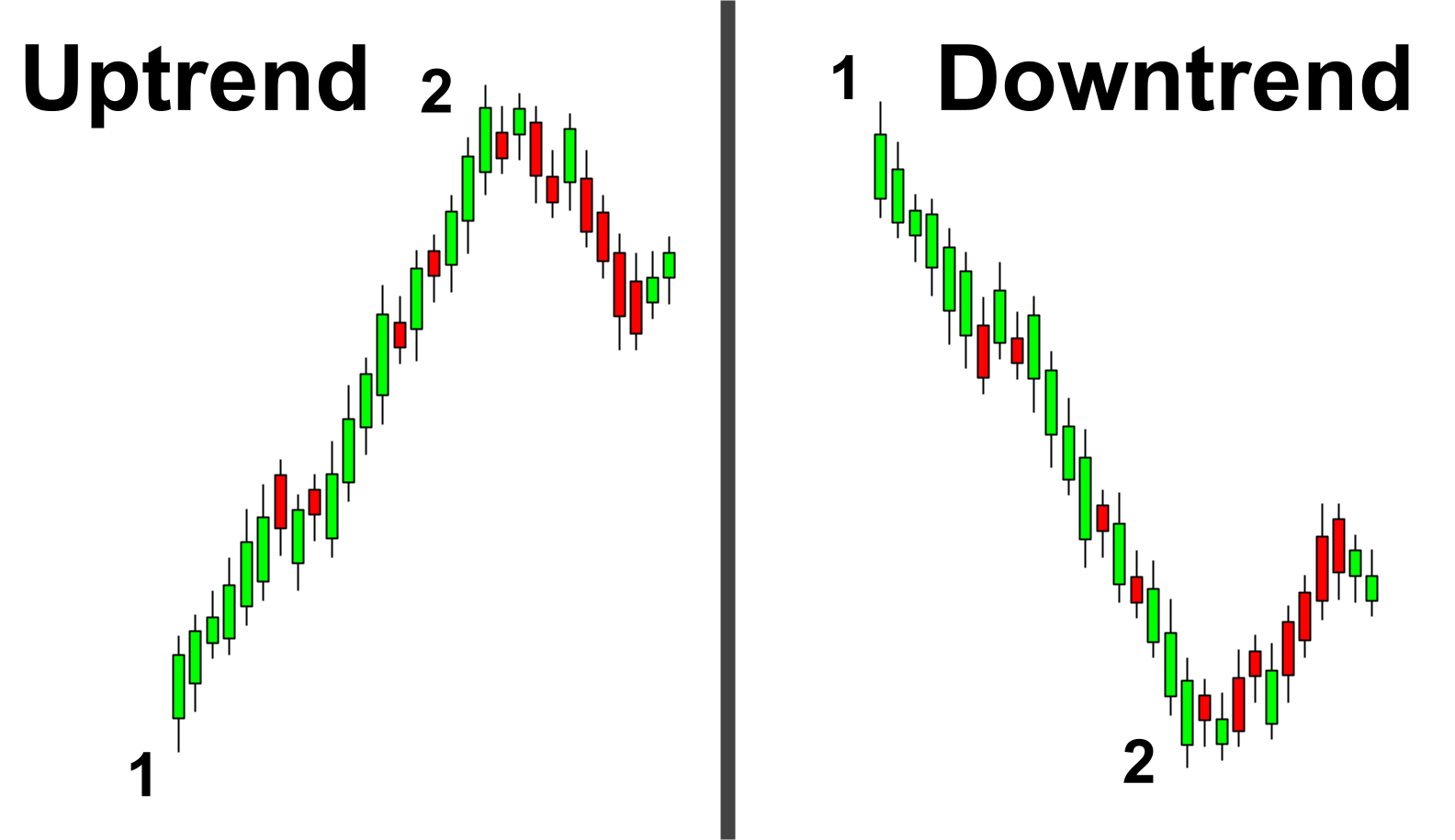1. First, find a completed trend to which you wish to apply the Fibonacci retracement
2. Find the charting tool called “Fibonacci retracement.” It’s available on plenty of charting software including TradingView.
3. Once you’ve activated the tool, make the first click at the beginning of the trend (1)
4. Make the second click at the end of the completed trend (2)
5. Customize the visible levels to be 23.6%, 38.2%, 61.8% and 78.6%

At this point, the Fibonacci retracement levels will appear on the chart. Traders will focus on these levels to identify reversal points within the subsequent correction.

## Using the Fibonacci Retracement Tool to Trade Crypto

The Fibonacci retracement tool is fairly simple to use and can be effective when trading crypto.

Step 1: First, find a completed trend. The tool can be applied to both uptrends and downtrends. The tool can also be applied to all chart time frames.

Step 2: Second, draw the Fibonacci retracement lines in the direction of the completed trend. For a completed uptrend, that means drawing the Fibonacci retracement from left to right in an upward direction. For a completed downtrend, draw the pattern from left to right, stopping at the end of the downtrend.

Step 3: Third, wait for the price to reverse near the four key levels. Focus on these key levels in order to anticipate a price reversal.

Step 4: Fourth, enter the trade in the direction of the original trend. A retracement of an uptrend means prices will correct lower. Then, time an entry into a bullish trade near one of the four key Fibonacci retracement levels. Some traders will blindly enter into the position at the retracement level. Other traders will wait for the price to react and break higher before entering long.

### Risk Management Technique

Once a good trading opportunity has been identified, traders will want to place a stop loss just below the swing low of a long trade, or just above the swing high of a short setup. Set up a strong risk-to-reward ratio by placing a target at least twice the distance to the stop loss.

The Fibonacci retracement tool is most powerful when used in tandem with other technical analysis indicators. For example, the Fibonacci level can send a buy or sell signal, but it may or may not reverse. It’s always best to confirm with indicators like Bollinger BandsStochastic RSI, or Ichimoku Cloud.

## Fibonacci Retracement Example for Bitcoin

There are many examples of Bitcoin reversing prices near a Fibonacci retracement level. The market is fractal, so you can find these examples within all chart time frames.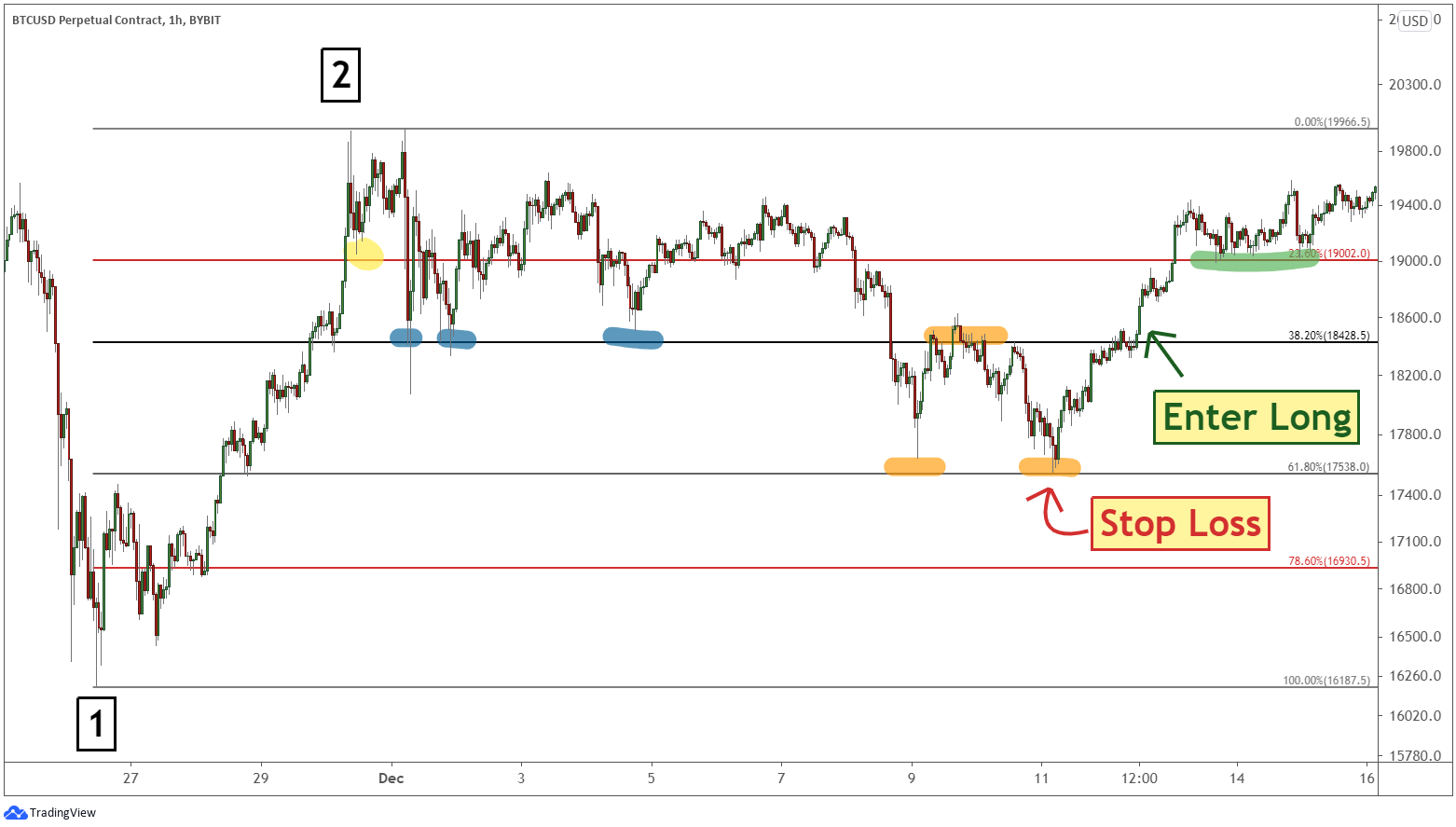After finding a low on November 26, 2020 (1), Bitcoin rallied about 23% to complete a trend on the 1-hour chart (2). When applying the tool from point 1 to point 2, these price zones are created on the chart.

In the chart above, Bitcoin begins a consolidation which immediately sees it correct to the 23.6% retracement level (yellow highlight), before rallying back up to meet the old high. The next corrective dip brings Bitcoin down to the 38.2% retracement level on three different occasions (blue highlights).

Bitcoin eventually breaks below the 38.2% retracement level and finds the next level of support near the 61.8% retracement level. Its price then bounces between the 61.8% and 38.2% Fibonacci retracement levels a couple of times (orange highlights).

After finding support at the 61.8% retracement level, Bitcoin resumes its uptrend, breaking above the 38.2% and 23.6% levels. Notice that once the 23.6% level has been broken, Bitcoin trades sideways on top of it (green highlight) before stretching to new all-time highs.

When Bitcoin prices break above the Fibonacci retracement level directly ahead, it can signal that the market is ready to turn higher. A trader will look to go long on a break above the next Fibonacci level with a stop loss just below the recent swing low. Look to target at least twice the distance to your stop loss.

### Bitcoin Trading Example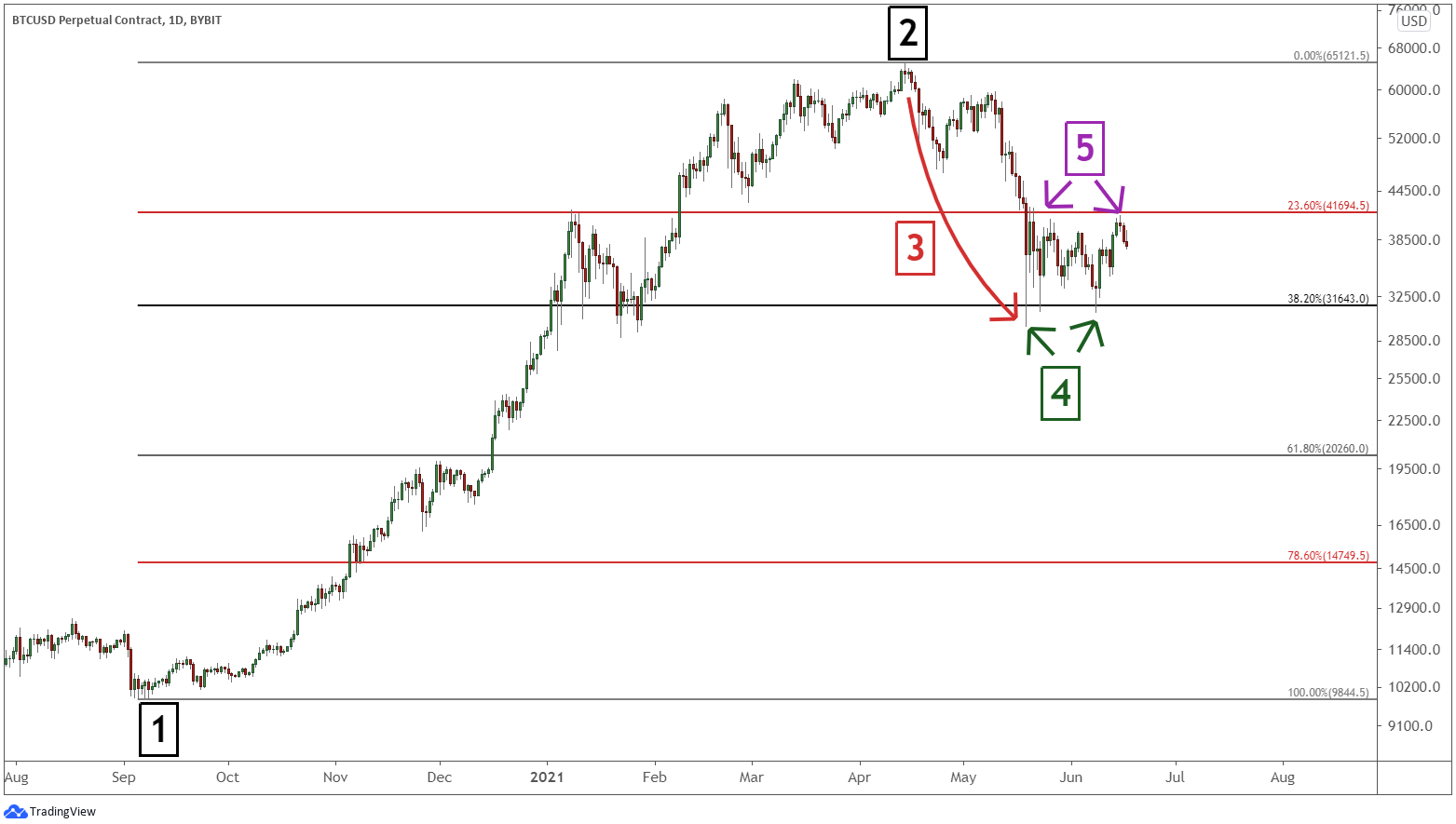Bitcoin started a new uptrend in September 2020 (1). This uptrend stalled and turned lower in April 2021 (2). Bitcoin’s price began going through a correction (3).

Bitcoin’s price found support at the 38.2% retracement level on two separate occasions (4). Frequently, you will see prices bounce between two Fibonacci retracement levels. In the chart above, Bitcoin has bounced between the 38.2% and 23.6% levels (points 4 and 5) as the bulls and bears battle. This example shows why Fibonacci retracement levels — both upward and downward — are important with respect to the market.

## Confirming Fibonacci With Other Technical Indicators

Fibonacci retracement is an effective tool by itself. However, there are other tools that traders typically incorporate in order to strengthen their signals.

The Fibonacci extension tool is a natural complement to retracements. The tool can help determine how far the current trend may travel.

Candlestick patterns and trend lines are useful with Fibonacci levels. If Bitcoin is approaching a retracement level, look for a candlestick pattern, such as the bullish engulfing, to confirm a resumption of the new bull trend.

Lastly, Elliott Wave Theory uses Fibonacci retracements quite a lot. Elliott Wave is an analytical method that studies the geometry of the market.

## Limitations

Fibonacci retracements are an effective tool, but they can be easily misunderstood.

First of all, if a trader draws them incorrectly, it can lead to the display of improper levels on the charts.

Secondly, smaller crypto markets may not have as much trading volume as a large market cap like Bitcoin. As a result, the Fibonacci retracement signals from a smaller market are not as reliable.

Finally, with four different levels to choose from, it is possible that the trader may focus on the wrong level, and, as a result, either miss a winning trade or take on a losing one.

## Final Thoughts

The Fibonacci retracement tool is one of the most powerful in a trader’s toolbox. It can help you identify hidden levels of support and resistance so that you can time your trades better. However, the tool is not foolproof. This tool is less effective on cryptocurrencies with a smaller market capitalization. In addition, it can be difficult to discern which level is the best one to use.